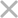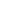400-023-17852021湖北文理学院专升本考试 《C语言程序设计》试题样卷
2021-11-08

《C语言程序设计》是2021湖北文理学院专升本计算机专业的专业课考试科目之一，另一个专业课考试科目是高等数学，公共课考试科目是大学英语，为了帮助大家了解C语言程序设计科目考什么内容？题型是怎么样的？今天给大家整理了C语言程序设计科目的样卷（该样卷为2021年湖北文理学院官方发出），C语言程序设计科目试卷题型均为客观题，全部是单项选择题，一共50道题，每小题2分，总分100分。（共50小题，每小题2分，共100分；在每小题列出的四个备选项中只有一个是符合题目要求的，请在答题卡上，将正确答案的代码填写在对应的题号处。）

1、下列C语言标识符中合法的是(   )。

A. *y    B. sum     C. int    D. %5f

2、下列可以正确表示字符型常量的是（  ）。

A. ’\t’   B. ”a”      C. ”\n”  D. 297

3．在C语言程序中，表达式8/5的结果是(   )。

A. 1.6    B. 1    C. 3   D. 0

4．若有int a=3,b=4；则条件表达式"a<b? a:b"的值是(  )。

A. 3    B. 4       C. 0      D. 1

5．在C语言中，认为(      )为逻辑"真" 。

A. true    B. 大于0的数     C. 非0整数     D. 小于0的数

6．设有语句“int a=2,b=3,c=-2,d=2;”,则逻辑表达式“a>0&&b&&c<0&&d>0”的值是（  ） 。

A. 1    B. 0       C. -1   D. 出错

7．C语言对嵌套if语句的规定是：else语句总是与（   ）配对。

A. 其之前最近的if        B. 第一个if

C. 缩进位置相同的if              D. 其之前最近的且尚未配对的if

8．设x为int型变量，则执行“x=10; x*=x;”后,x的值为（  ）

A. 10    B. 20       C. 100   D. 0

9．若有“int a=1,x=1;”,则循环语句“while(a<10) x++; a++;”执行（ ）

A. 无限次   B. 不确定      C. 10次      D. 9次

10.设有说明char c;int i;float f;则表达式c*i+f*f值的数据类型为( )。

A. float   B. char   C. int   D. double

11. 下列表达式为关系表达式的是_______。(设其中a为一整型变量)

A. 3+4*5     B. a==10       C. a>0?a:-a    D. a=1

12．不属于字符型常量的是_______。

A. ‘A’    B. '\0x1f'     C. “A”     D. '\123'

13．下列属于整型常量的是_______。

A. 12       B. 12.0        C. -12.0    D. 10E10

14．下面不正确的赋值语句是_______。（设变量已定义有初值）

A. a=++a       B. a=b++=10      C. a+=b       D. a=1>‘a’

15．下面哪个可作为用户自定义变量_______。

A. void     B. at        C. 529p       D. w3-q_9

16．int a,b,m,n;它们的初值依次为1、2、3、4，经运算m=(a<=b&&(n=m<n))后，m、n的值是_______。

A. 1    1       B. 1   0      C. 0    1      D.  0   0

17．若x=1,y=4 则y*=x+5; y的值为_______。

A.  24        B.  9       C.  20      D. 30

18．循环语句 for (x=0; x<4;x++)  y++; 的循环体执行_______。

A. 5次      B. 4次         C. 3次    D. 2次

19．表达式int  x=1; 执行x=(x<1？3:2);后，x的值为_______。

A. 3         B. 2           C. 1         D. 0

20．以下说法中正确的是_______。

A. C语言程序总是从第一个定义的函数开始执行。

B. 在C语言程序中，要调用的函数必须在main( ) 函数中定义。

C. C语言程序总是从main( ) 函数开始执行。

D. C语言程序中的main( ) 函数必须放在程序的开始部分。

21．下列语句中，能正确输出26个英文字母的是______。

A. for(a='a';a<='z';printf("%c",++a));     B. for(a='a';a<='z';) printf("%c",a);

C. for(a='a';a<='z';printf("%c",a++));     D. for(a='a';a<='z';printf("%c",a));

22．当执行以下程序段时，______。

int a=1;

do{  a=a*a;  }while(!a);

A. 循环体将执行1次                          B. 循环体将执行2次

C. 循环体将执行无限次                        D. 系统将提示有语法错误

23．以下程序段，while循环中循环体的执行次数是______。

int  k=1;  while(k!=0) k++;

A. 无限次        B. 有限次         C. 一次也不执行      D. 执行1次

24．以下程序段，循环体sum++的执行次数是______。

int  i. j, sum=0;

for(i=1;i<5;i++)

for( j=1;j<=i; j++) sum++;

A. 5次             B. 10次            C. 15次              D. 20次

25．以下正确的函数定义是______。

A. double  fun(int  x,int  y)  {  z=x+y;   return z;  }

B. double  fun(int  x, y)    {  int z;   return z;  }

C. fun (int  x, int  y)    {  double z ; z=x+y;  return z;  }

D. double  fun(int  x, int  y)  {  double z;    return z;  }

26.下列四组选项中, 全部是C 语言关键字的选项是( )。

A. define IF type           B. getc char printf

C. include scanf case          D. while switch else

27.int x=0,y=0;while(x<10){if(x%2)continue;y++;x++;};该程序执行后 y的值是（ ）。

A. 0                 B. 5             C. 10                D. 程序陷入死循环

28.在位运算中,操作数每右移一位,其结果相当于( )。

A. 操作数乘以2            B. 操作数除以2

C. 操作数乘以16        D. 操作数除以16

29.关于指针概念说法不正确的是( )。

A. 一个指针变量只能指向同一类型变量

B. 一个变量的地址称为该变量的指针

C. 只有同一类型变量的地址才能放到指向该类型变量的指针变量之中

D. 一个变量的地址可以被改变

30.若有int *p=(int *)malloc(sizeof(int));则向申请到内存空间存入整数123的语句为( )。

A. scanf("%d",p);           B. scanf("%d",&p);

C. scanf("%d",*p);           D. scanf("%d",**p);

31.关于return语句,下列错误的说法是( )。

A. return语句可以不带返回值        B. 必须在每个函数中出现

C. 可以在同一个函数中出现多次        D. 一旦被执行，函数立即结束

32.若定义int k=7,x=12; 则能使值为3的表达式是( )。

A. x%=(k%=5)    B. x%=(k-k%5)      C. x%=k-k%5    D. (x%=k)-(k%=5)

33.关于C 语言中的实参与形参，以下正确的说法是( )。

A. 实参和与其对应的形参各占用独立的存储单元

B. 实参和与其对应的形参共占用一个存储单元

C. 只有当实参和与其对应的形参同名时才共占用存储单元

D. 形参是虚拟的,不占用存储单元

34.设有说明char w;int x;float y;double z;则表达式w*x+z-y值的数据类型为( )。

A. float      B. char         C. int      D. double

35.以下各选项拟说明一种新的类型名，其中正确的是( )。

A. typedef v1 int;           B. typedef v2=int;

C. typedef int v3;           D. typedef v4: int;

36.执行下面程序后，输出结果是( )。

int max(int x, int y)

{ int z; if(x>y) z=x; else z=y; return z; }

int main()

{ int a=45,b=27,c=0; c=max(a,b); printf("%d\n",c); return 0; }

A. 45       B. 27      C. 18    D. 72

37.下面有关for 循环的正确描述是 ()

A. for 循环只能用于循环次数已经确定的情况

B. for 循环是先执行循环体语句,后判断表达式

C. 在for 循环中,不能用break 语句跳出循环体

D. for 循环的循环体语句中,可以包含多条语句,但必须用花括号括起来

38.以下选项是字符串常量的是（）。

A. “\n”       B. ‘\t'     C. ‘\c'       D. '\\'

39.char h; char *s=&h;可将字符‘H’通过指针存入变量h中的语句是()。

A. s=H;       B. *s='H';        C. s=H;       D. s='H'

40.假设0≤i<10，以下语句中不是对a数组元素的正确引用的是（）。

int a={0,1,2,3,4,5,6,7,8,9,};

int  *p = a;

A. a[i]       B. *p      C. p        D. *(p+1)

41.C语言程序从(    )开始执行。

A. 程序中第一条可执行语句         B. 程序中第一个函数

C. 程序中的main函数          D. 包含文件中的第一个函数

42.根据已定义的两条C语句：struct person { char name; int age; };

A. printf(" %c\n"，class.name);    B. printf(" %c\n"，class.name);

C. printf(" %c\n"，class.name); D. printf(" %c\n"，class.name);

43. 在C语言程序中，表达式5%2的结果是（    ）。

A. 2.5       B. 2           C. 1         D. 3

44. 设整型变量 a=2，则执行下列语句后，浮点型变量b的值不为0.5的是（    ）。

A. b=1.0/a      B. b=(float)(1/a)   C. b=1/(float)a   D. b=1/(a*1.0)

45. C语言中函数返回值的类型是由（    ）决定的。

A. 函数定义时指定的类型        B. return语句中的表达式类型

C. 调用该函数时的实参的数据类型      D. 形参的数据类型

46. 在函数调用时，以下说法正确的是（    ）。

A. 函数调用后必须带回返回值            B. 实际参数和形式参数可以同名

C. 实际参数不能是变量                  D. 主调函数和被调函数总是在同一个文件里

47.putchar函数可以向终端输出一个（    ）。

A. 整型变量表达式   B. 实型变量值      C. 字符串        D. 字符

48.若有条件表达式(exp)?a++:b--,则以下表达式中能完全等价于表达式(exp)的是（    ）。

A. exp==0        B. exp!=0       C. exp==1       D. exp!=1

49.作为函数fopen( )的第一个参数，格式正确的选项是（  ）。

A. c:user\text.txt            B. c:\user\text.txt

C. "c:\user\text.txt"            D. "c:\\user\\text.txt"

50.fgetc函数的作用是从指定文件读入一个字符，该文件的打开方式必须是（  ）。

A. 只写             B. 追加            C. 读或读写          D. 答案 B 和 C 都正确

* 姓名
* 手机
* 所在学校